In Computer Methods in Applied Mechanics and Engineering, Vol. 365, pp. 112984, 2020
DOI: 10.1016/j.cma.2020.112984

## Abstract

In this paper we present a stabilized FIC-FEM formulation for the multidimensional transient advection-diffusion-absorption equation. The starting point is the non-local form of the governing equations for the multidimensional transient advection-diffusion-absorption problems obtained via the Finite Increment Calculus (FIC) procedure. The FIC governing equations have a residual form that introduces a characteristic length vector that depends on streamline, absorption and shock capturing stabilization parameters, as well as on a characteristic element size that ensures a stabilized numerical solution using a standard Galerkin FEM. The value of the stabilization parameters is obtained as an extension of the steady-state form. The accuracy of the FIC-FEM formulation is verified in the solution of several transient advection-diffusion-absorption problems using regular meshes of 3-noded triangles and 4-noded quadrilaterals.

Keywords: Advection-diffusion-absorption, transient solution, multidimensional, Finite element method, Finite increment calculus, FIC

## 1. Introduction

The numerical solution of the advection-diffusion-absorption equation by the Galerkin FEM can exhibit oscillations due to a number of reasons. For the stationary convection-dominated case global oscillations in the analysis can be found, as well as local Gibbs oscillations along the characteristic layers for 2D and 3D problems. Gibbs oscillations near the Dirichlet boundaries and in regions where the source term has an irregular distribution can also appear for stationary absorption-dominated problems. For transient problems dispersive oscillations can appear when the initial solution and/or the distributed source term are non regular [12,68].

A number of numerical procedures has been derived in recent decades aiming to overcome the above limitations of Galerkin FEM for solving advection-diffusion-absorption problems. The goal in all cases has been to obtain “stabilized” numerical solution that are free of spurious oscillations and have a physical meaning. Among the many procedures we can mention the family of streamline-upward Petrov-Galerkin (SUPG) methods. The original SUPG methods for advection-diffusion problems [5,25,26,33,35] where enhanced with the addition of non linear shock-capturing terms aiming to control the Gibbs oscillations across internal/boundary layers for advection-diffusion problems [7,10,13,14,20,32,34,36,51]. Other families of stabilized methods for this type of problems were based on Galerkin Least Squares (GLS) , Variational Multiscale (VMS)  and Characteristic split procedure [66,68]. Many of these methods are reviewed in .

Control over the dispersive oscillations for transient advection-diffusion problems via SUPG methods and space-time FEM were reported in  and , respectively.

Extensions of the SUPG method for advection-diffusion-reaction problems were reported in [6,29,64]. Other stabilization techniques for these problems were based in the GLS method [17,21], the addition of internal bubbles [1,2,3,4,18,19] and on variations of VMS procedure [9,22,23,24,63]. A review of some of these methods is presented in .

Oñate et al. [52,55] derived a non linear stabilization procedure for the steady state advection-diffusion-absorption problem using a single stabilization parameter via a Finite Increment Calculus (FIC) approach. Oñate and Felippa  used the variational FIC method for obtaining exact nodal solutions for 1D diffusion-reaction problems (including Helmholtz problems) using also a single linear stabilization parameter.

The homogeneous steady advection-diffusion-reaction equation has typically two fundamental solutions. This has lead the way to the derivation of linear stabilized methods that provide nodally exact solutions in 1D using two stabilization parameters via internal bubbles , SUPG  and VMS  procedures.

A particular class of two-parameter stabilized models for 1D and multidimensional steady and transient advection-diffusion production problems using the FIC approach and the FEM vas presented by Nadukandi, Oñate and García-Espinosa [37,38].

Starting from a different perspective, but still within the FIC framework, Oñate, Miquel and Nadukandi  presented a FIC-FEM formulation for the 1D steady-state and transient advection-diffusion-absorption equations using two linear stabilization parameters. The FIC-FEM formulation yielded exact nodal solution for 1D steady-state problems using regular meshes of 2-noded linear elements. Very accurate results were also obtained in  for 1D stationary problems solved with irregular meshes, as well as for 1D transient problems. The FIC-FEM formulation presented in  was extended by the same authors in  to multidimensional steady-state advection-diffusion-absorption problems. Good results were obtained for the problems analyzed using linear 3-noded triangles and bilinear 4-noded quadrilaterals.

In this work we extend to the multidimensional transient case the 1D FIC-FEM formulation presented in . The lay-out of the paper is the following. In the next section we formulate the FIC form of the equations governing multidimensional transient convection-diffusion-absorption problems. The finite element discretization is then presented. The stabilization parameters are obtained as an extension of the expression for the stationary case . The accuracy of the multidimensional transient FIC-FEM formulation is verified in the solution of a number of 2D transient advection-diffusion-absorption problems using uniform meshes of 3-noded triangles and 4-noded quadrilateral elements. Accurate solutions are obtained in all cases.

## 2. The multidimensional transient advection-diffusion-reaction problem

### 2.1 Governing equations

Transport balance

The transport balance equation in a domain of area/volume ${\textstyle \Omega }$ can be expressed as

 $r_{t}=0\quad {\hbox{in }}\Omega$
(1.a)

with

 $r_{t}:=\rho c\left({\frac {\partial \phi }{\partial t}}+\mathbf {v} ^{T}{\boldsymbol {\nabla }}{\phi }\right)-{\boldsymbol {\nabla }}^{T}{\boldsymbol {D}}{\boldsymbol {\nabla }}\phi +s\phi -Q$
(1.b)

For 3D problems,

 $\mathbf {v} =[v_{1}~,~v_{2}~,~v_{3}]T\quad ,\quad {\boldsymbol {D}}=\left[{\begin{array}{ccc}k_{1}&0&0\\0&k_{2}&0\\0&0&k_{3}\end{array}}\right]\quad ,\quad {\boldsymbol {\nabla }}=\left[{\frac {\partial }{\partial x_{1}}}~,~{\frac {\partial }{\partial x_{2}}}~,~{\frac {\partial }{\partial x_{3}}}\right]^{T}$
(2)

In Eqs.(1.a)–(1.b) ${\textstyle \phi }$ is the transported variable (i.e., the temperature in a heat transfer problem or the concentration in a mass transfer problem), ${\textstyle v_{i}}$ is the ${\textstyle i}$th component of the velocity vector ${\textstyle {\mathbf {} v}}$; ${\textstyle \rho ,c}$ and ${\textstyle k_{i}}$ are the density, the specific flux parameter and the conductivity of the material along the ${\textstyle i}$th global direction, respectively and ${\textstyle s}$ is the reaction parameter. In the following, and unless otherwise specified, we will assume that the velocity field is solenoidal and that the problem parameters (${\textstyle \rho ,c,k,s}$) are constant over the analysis domain ${\textstyle \Omega }$.

Boundary conditions

 $\phi -\phi ^{p}=0\quad {\hbox{on }}\Gamma _{\phi }$
(3)

 $r_{\scriptscriptstyle \Gamma }=0\quad {\hbox{on }}\Gamma _{q}$
(4)

with

 $r_{\Gamma }:=-q_{n}+q_{n}^{p}$
(5)

where

 $q_{n}={\boldsymbol {q}}^{T}{\boldsymbol {n}}\quad ,\quad {\boldsymbol {q}}=-{\boldsymbol {D}}{\boldsymbol {\nabla }}\phi$
(6)

In Eqs.(3)–6 ${\textstyle \phi ^{p}}$ and ${\textstyle q^{p}}$ are the prescribed values of the transported variable and the outgoing diffusive flux at the Dirichlet and Neumann boundaries ${\textstyle \Gamma _{\phi }}$ and ${\textstyle \Gamma _{q}}$, respectively, with ${\textstyle \Gamma _{\phi }\cup \Gamma _{q}=\Gamma }$, ${\textstyle \Gamma }$ being the total boundary of the domain and n is the unit vector normal to the boundary.

The definition of the problem is completed with the initial conditions

 $\phi ({\boldsymbol {x}},t_{0})=\phi _{0}({\boldsymbol {x}})$
(7)

where ${\textstyle \phi _{0}({\boldsymbol {x}})}$ is the value of the transported variable at time ${\textstyle t=t_{0}}$.

In this work we will consider cases for which ${\textstyle s\geq 0}$ only. This includes the following particular problems:

• Advection-diffusion-absorption (${\textstyle |{\boldsymbol {v}}|\not =0}$, ${\textstyle K\not =0}$, ${\textstyle s>0}$).
• Advection-diffusion (${\textstyle |{\boldsymbol {v}}|\not =0}$, ${\textstyle K\not =0}$, ${\textstyle s=0}$).
• Diffusion-absorption (${\textstyle |{\boldsymbol {v}}|=0}$, ${\textstyle K\not =0}$, ${\textstyle s>0}$).
• Advection-absorption (${\textstyle |{\boldsymbol {v}}|\not =0}$, ${\textstyle K=0}$, ${\textstyle s>0}$).

In the above ${\textstyle K}$ is the average difussion given by ${\textstyle K=\left[{\frac {1}{n_{d}}}\sum \limits _{i=1}^{n_{d}}(k_{i})^{2}\right]^{1/2}}$, where ${\textstyle n_{d}}$ is the number of space dimensions (i.e. ${\textstyle n_{d}=2}$ for 2D problems).

### 2.2 Finite increment calculus (FIC) expressions

The governing equations 1.a and 1.b and the boundary conditions 3-6 are expressed using the FIC theory as .

Transport balance

 $r_{t}-{\underline {{\frac {1}{2}}{\boldsymbol {h}}^{T}{\boldsymbol {\nabla }}r_{t}}}{\frac {1}{2}}{\boldsymbol {h}}^{T}{\boldsymbol {\nabla }}r_{t}=0\qquad {\hbox{in }}\Omega$
(8)

with ${\textstyle {\boldsymbol {h}}=[h_{1},h_{2},h_{3}]^{T}}$ in 3D.

Boundary conditions

 $\phi -\phi ^{p}=0\quad {\hbox{on }}\Gamma _{\phi }$
(9.a)

 $r_{\Gamma }+{\underline {{\frac {1}{2}}h_{n}r_{t}}}{\frac {1}{2}}h_{n}r_{t}=0\quad {\hbox{on }}\Gamma _{q}\quad ,\quad {\hbox{with }}h_{n}={\boldsymbol {h}}^{T}{\boldsymbol {n}}$
(9.b)

Eqs.(8) and (9.b) are obtained by expressing the balance of fluxes in an arbitrary prismatic space domain of size ${\textstyle h_{1}\times h_{2}\times h_{3}}$ within the global problem domain and at the Neumann boundary, respectively. The distances ${\textstyle h_{i}}$ are termed characteristic lengths of the FIC method. The variations of the transported variable within the balance domain are approximated by Taylor series expansions retaining one order higher terms than in the infinitesimal theory . This higher order expansions lead to the underlined terms in Eqs.(8) and (2.2). These terms lead naturally to stabilized numerical schemes.

Note that as the characteristic length vector ${\textstyle {\boldsymbol {h}}}$ tends to zero the FIC governing equations gradually recover the standard infinitesimal form, giving in the limit (for ${\textstyle \mathbf {h} =\mathbf {0} }$) ${\textstyle r_{t}=0}$ in ${\textstyle \Omega }$ and ${\textstyle r_{\scriptscriptstyle \Gamma }=0\quad {\hbox{on }}\Gamma _{q}}$.

The stability and accuracy of the numerical solution depends on the components of the characteristic length vector ${\textstyle {\boldsymbol {h}}}$. At the discretization level the length of ${\textstyle {\boldsymbol {h}}}$ is usually expressed as a proportion of a typical grid dimension .

The FIC equations are the starting point for deriving different stabilized numerical methods. Combining the FIC equations with the Galerkin FEM leads to the so-called FIC-FEM procedure that has been successfully applied to the solution of many problems in convective transport, fluid and solid mechanics such as advection-diffusion [40,42,43,51], diffusion-absorption and Helmholtz , advection-diffusion-absorption [52,55], advection-diffusion-reaction , incompressible fluid flow [53,54,56,58,60], fluid-structure-interaction [45,49,57], particle-laden flows and standard and incompressible solid mechanics [47,50,59]. The FIC approach has also been applied to the solution of a variety of problems in mechanics using the meshless finite point method [39,41,44,46].

## 3. Definition of the characteristic length vector

The characteristic length vector ${\textstyle {\boldsymbol {h}}}$ is designed so that the expression for all the stabilization matrices and vectors reduce to those given for the 1D case as reported in .

With this objective in mind the following expression for the characteristic length vector has been chosen in this work

 ${\boldsymbol {h}}={\boldsymbol {h}}_{v}+{\boldsymbol {h}}_{r}+{\boldsymbol {h}}_{sc}$
(10)

The characteristic length vectors in the r.h.s. of Eq.10 are defined as follows.

Streamline characteristic length vector, hv

In Eq.10 ${\textstyle {\boldsymbol {h}}_{v}}$ is a length vector along the velocity direction defined as

 ${\boldsymbol {h}}_{v}=\alpha _{v}l_{v}{\frac {\mathbf {v} }{|\mathbf {v} |}}=\alpha _{v}l_{v}{\hat {\boldsymbol {v}}}$
(11)

where ${\textstyle \alpha _{v}}$ is a streamline stabilization parameter, ${\textstyle l_{v}}$ is a characteristic element dimension and ${\textstyle {\hat {\boldsymbol {v}}}}$ is a unit velocity vector.

Absorption characteristic length vector, hr

In Eq.10 ${\textstyle {\boldsymbol {h}}_{r}}$ is a characteristic length vector induced by absorption effects and defined as

 ${\boldsymbol {h}}_{r}={\boldsymbol {H}}_{r}{\boldsymbol {\nabla }}\phi \qquad {\hbox{with}}\quad {\boldsymbol {H}}_{r}={\frac {2sgn(r_{t})}{r_{s}}}\left[{\boldsymbol {D}}_{s}+\alpha _{r}D{\hat {\boldsymbol {v}}}{\hat {\boldsymbol {v}}}^{T}\right]$
(12.a)

where ${\textstyle \alpha _{r}}$ is an absorption stabilization parameter, ${\textstyle {D}={\hat {\boldsymbol {v}}}^{T}{\boldsymbol {D}}{\hat {\boldsymbol {v}}}}$ and ${\textstyle r_{s}}$ is the space residual defined as

 $r_{s}:=r_{t}-\rho c{\partial \phi \over \partial t}$
(12.b)

Matrix ${\textstyle {\boldsymbol {D}}_{s}}$ in Eq.12.a is defined for different element types as follows.

##### 3-noded triangles and 4-noded tetrahedra:

 ${\boldsymbol {D}}_{s}={\frac {s}{(n+1)}}\sum \limits _{i=1}^{n}{\boldsymbol {l}}_{i}{\boldsymbol {l}}_{i}^{T}$
(13)

where ${\textstyle {\boldsymbol {l}}_{i}}$ is the vector joining the baricenter of the element and the ${\textstyle i}$th node, and ${\textstyle n}$ is the number of nodes of the element.

The diffusion introduced by matrix ${\textstyle {\boldsymbol {D}}_{s}}$ takes care of the instabilities induced by the irregularity of the triangular mesh near boundaries that develop parabolic layes .

##### Any other element: Dₛ=

Shock capturing characteristic length vector hsc

In Eq.10 ${\textstyle {\boldsymbol {h}}_{sc}}$ is a shock-capturing characteristic length vector in the direction of the gradient of the solution. This vector accounts for the Gibbs oscillations across characteristic internal/boundary layers for advection-diffusion problems. It is defined as

 ${\boldsymbol {h}}_{sc}={h}_{sc}{\widehat {{\boldsymbol {\nabla }}\phi }}$
(14.a)

where ${\textstyle {\widehat {{\boldsymbol {\nabla }}\phi }}={\frac {{\boldsymbol {\nabla }}\phi }{|{\boldsymbol {\nabla }}\phi |}}}$ is the unit gradient vector, and

 ${h}_{sc}=(1-\beta ^{2})\left[l_{sc}sgn(r_{s})-{\frac {2|{\boldsymbol {\nabla }}\phi |}{r_{s}}}({\boldsymbol {D}}+{\boldsymbol {D}}_{s}):({\boldsymbol {I}}-{\hat {\boldsymbol {v}}}{\hat {\boldsymbol {v}}}^{T})\right]$
(14.b)

In Eq.14.b ${\textstyle \beta }$ is a non linear parameter that depends on the angle ${\textstyle \theta }$ between the velocity vector and the gradient vector. It is defined as

 $\beta =\left\{{\begin{array}{lll}1&{\hbox{ if }}&\theta <\theta _{c}\\\displaystyle {\hat {\boldsymbol {v}}}^{T}{\widehat {{\boldsymbol {\nabla }}\phi }}&{\hbox{ if }}&\theta \geq \theta _{c}\end{array}}\right.$
(14.c)

where ${\textstyle \theta _{c}}$ is a critical angle. In this work we have taken ${\textstyle \theta _{c}=20^{\circ }}$.

The parameter ${\textstyle \beta }$ controls the amount of shock-capturing nonlinear diffusion active at any point of the domain . When the gradient vector is parallel to the velocity vector then ${\textstyle \beta =1}$, ${\textstyle h_{sc}}$ vanishes and the linear stabilization terms suffice to diminish spurious numerical oscillations about the layers. The term ${\textstyle 1-\beta ^{2}}$ in Eq.14.b gradually increases the magnitude of the shock-capturing term from zero, when the gradient vector is aligned to velocity vector, to a maximum value when the layer gradient is orthogonal to the velocity.

## 4. FIC-FEM formulation for the multidimensional transient advection-diffusion-absorption problem

### 4.1 Weighted residual form of the FIC equations

The weighted residual form of the FIC governing equations 8 and 2.2 is written as

 $\int _{\Omega }W(r_{t}-{\frac {1}{2}}{\boldsymbol {h}}^{T}{\boldsymbol {\nabla }}r_{t})d\Omega +\int _{\Gamma _{q}}W(-q_{n}+q_{n}^{p}+{\frac {1}{2}}h_{n}r_{t})d\Gamma =0$
(15)

where ${\textstyle W}$ are arbitrary weighting functions.

Integrating by parts the FIC term in the first integral of 15 gives

 $\int _{\Omega }\left(W+{\frac {1}{2}}({\boldsymbol {\nabla }}^{T}W){\boldsymbol {h}}\right)r_{t}d\Omega +\oint _{\Gamma _{q}}W(-q_{n}+q_{n}^{p})d\Gamma =0$
(16)

Note that the FIC term has vanished from the boundary integral, as it usual in the FIC-FEM approach [40,48].

Let us substitute the expression for the characteristic vector ${\textstyle {\boldsymbol {h}}}$ of Eq.10 into Eq.16. This gives (using Eqs.11, 12.a and 14.a)

 $\int _{\Omega }\left[Wr_{t}+{\frac {1}{2}}({\boldsymbol {\nabla }}^{T}W)\left(\alpha _{v}l_{v}{\hat {\boldsymbol {v}}}+{\boldsymbol {H}}_{r}{\boldsymbol {\nabla }}\phi +{h}_{sc}{\frac {{\boldsymbol {\nabla }}\phi }{|{\boldsymbol {\nabla }}\phi |}}\right)r_{t}\right]d\Omega +\oint _{\Gamma _{q}}W(-q_{n}+q_{n}^{p})d\Gamma =0$
(17)

The final step is the integration by parts of the diffusive term in the expression of ${\textstyle r_{t}}$ in the first term of the first integral of Eq.17. This gives, after replacing the definition of ${\textstyle {\boldsymbol {H}}_{r}}$ in Eq.17 and grouping some terms, the following expression for the weak variational form of the FIC governing equations

 $\displaystyle \int _{\Omega }\left[\rho c{\bar {W}}{\frac {\partial \phi }{\partial t}}+\rho cW{\boldsymbol {v}}^{T}{\boldsymbol {\nabla }}\phi +({\boldsymbol {\nabla }}^{T}W){\boldsymbol {D}}_{T}{\boldsymbol {\nabla }}\phi +Ws\phi +{\frac {1}{2}}({\boldsymbol {\nabla }}^{T}W){\boldsymbol {h}}_{v}s\phi \right]d\Omega +$ $\displaystyle \int _{\Omega }{\frac {1}{2}}({\boldsymbol {\nabla }}^{T}W){\boldsymbol {h}}\left[-{\boldsymbol {\nabla }}^{T}({\boldsymbol {D}}{\boldsymbol {\nabla }}\phi )-Q\right]d\Omega -\int _{\Omega }WQd\Omega +\oint _{\Gamma _{q}}Wq_{n}^{p}d\Gamma =0$
(18)

The space weighting function ${\textstyle {\bar {W}}}$ is given by

 ${\bar {W}}=W+{\frac {1}{2}}{\boldsymbol {h}}_{v}^{T}{\boldsymbol {\nabla }}W$
(19)

The expression of the total diffusivity matrix ${\textstyle {\boldsymbol {D}}_{T}}$ in Eq.18 is

 $\displaystyle {\boldsymbol {D}}_{T}={\boldsymbol {D}}+\alpha _{v}{\boldsymbol {D}}_{v}+{\boldsymbol {D}}_{s}+\alpha _{r}{D}{\hat {\boldsymbol {v}}}{\hat {\boldsymbol {v}}}^{T}+{\boldsymbol {D}}_{sc}{\boldsymbol {I}}$
(20)

where ${\textstyle {\boldsymbol {D}}}$ and ${\textstyle {\boldsymbol {D}}_{s}}$ are defined in Eqs.2 and 13, respectively, I is the unit matrix and

 ${\boldsymbol {D}}_{v}\!\!\!\!=\!\!{\frac {l_{v}}{2}}{\hat {\boldsymbol {v}}}{\boldsymbol {v}}^{T}$ (21.a) ${\boldsymbol {D}}_{sc}\!\!\!\!=\!\!\left({\frac {1}{2}}l_{s_{c}}{\frac {|r_{t}|}{|{\boldsymbol {\nabla }}\phi |}}-({\boldsymbol {D}}+{\boldsymbol {D}}_{s}):({\boldsymbol {I}}-{\hat {\boldsymbol {v}}}{\hat {\boldsymbol {v}}}^{T})\right)(1-\beta ^{2})$ (21.b)

### 4.2 FIC-FEM equations

We interpolate the transported variable ${\textstyle \phi }$ in the standard FEM fashion over a mesh of elements with ${\textstyle n}$ nodes [67,68] as

 $\phi \simeq {\hat {\phi }}=\sum \limits _{i=1}^{n}N_{i}\phi _{i}$
(22)

where ${\textstyle N_{i}}$ are the space shape functions and ${\textstyle \phi _{i}}$ the nodal variables.

Introducing Eq.22 into the FIC variational form 18 and using a Galerkin approach (${\textstyle W_{i}=N_{i}}$) gives the final system of discretized equations as

 $\mathbf {M} {\dot {\boldsymbol {\phi }}}+[\mathbf {K} +\mathbf {C} +\mathbf {S} ]{\boldsymbol {\phi }}={\boldsymbol {f}}$
(23)

where ${\textstyle {\boldsymbol {\phi }}=[\phi _{1},\phi _{2},\cdots ,\phi _{N}]^{T}}$ is the vector of nodal unknowns, with ${\textstyle N}$ being total number of nodes in the mesh.

The rest of matrices and vector ${\textstyle {\boldsymbol {f}}}$ in Eq.23 are obtained in the standard FEM fashion by assembling the element contribution given by

 $\mathbf {M} _{ij}^{e}=\int _{\Omega ^{e}}\rho cN_{i}N_{j}d\Omega +\int _{\Omega ^{e}}\rho c{\frac {1}{2}}({\boldsymbol {\nabla }}^{T}N_{i}){\boldsymbol {h}}_{v}N_{j}d\Omega$ (24) $\mathbf {K} _{ij}^{e}=\int _{\Omega ^{e}}({\boldsymbol {\nabla }}^{T}N_{i}){\boldsymbol {D}}_{T}{\boldsymbol {\nabla }}N_{j}d\Omega -\int _{\Omega ^{e}}{\frac {1}{2}}({\boldsymbol {\nabla }}^{T}N_{i}){\boldsymbol {h}}{\boldsymbol {\nabla }}^{T}({\boldsymbol {D}}{\boldsymbol {\nabla }}N_{j})d\Omega$ (25) $\mathbf {C} _{ij}^{e}=\int _{\Omega ^{e}}\rho cN_{i}{\boldsymbol {v}}^{T}{\boldsymbol {\nabla }}N_{j}d\Omega$ (26) $\mathbf {S} _{ij}^{e}=\int _{\Omega ^{e}}sN_{i}N_{j}d\Omega +\int _{\Omega ^{e}}{\frac {1}{2}}({\boldsymbol {\nabla }}^{T}N_{i}){\boldsymbol {h}}_{v}sN_{j}d\Omega$ (27) ${\boldsymbol {f}}_{i}^{e}=\int _{\Omega ^{e}}\left(N_{i}+{\frac {1}{2}}({\boldsymbol {\nabla }}^{T}N_{i}){\boldsymbol {h}}\right)Qd\Omega -\oint _{\Gamma _{q}^{e}}N_{i}q_{n}^{p}d\Gamma$ (28)

We note that the second integral in Eq.25 vanishes for linear finite element approximations, such as those used in this work.

Good results have been obtained for the solution of the problems presented in this paper using a lumped form of matrix ${\textstyle {\boldsymbol {M}}}$. An exception is the problem of the evolution of a concentration field presented in Section 7.6 for which using the consistent form of matrix ${\textstyle {\boldsymbol {M}}}$ is essential to reduce excessive diffusion.

## 5. Transient solution scheme

### 5.1 Implicit time integration scheme

We discretize in time the system of equations (23) using a Generalized Trapezoidal rule [11,67]. The solution for the nodal values at a time instant is found using an incremental iterative strategy as

 ${}^{i}{\boldsymbol {H}}^{n}\Delta {\boldsymbol {\phi }}=-{}^{i}{\boldsymbol {r}}_{t}^{n}$
(29)

where ${\textstyle \Delta {\boldsymbol {\phi }}}$ is the increment of the nodal variables, ${\textstyle \theta }$ is a non dimensional time parameter (${\textstyle 0.5<\theta \leq 1}$ is required for the integration scheme to be unconditionally stable [11,67,68]), ${\textstyle (\cdot )^{n}}$ denotes values at time ${\textstyle t=t_{n}}$, ${\textstyle {}^{i}(\cdot )}$ denotes values at the ${\textstyle i}$th iteration and

 ${}^{i}{\boldsymbol {H}}^{n}={\frac {1}{\theta \Delta t}}{\boldsymbol {M}}+{}^{i}{\boldsymbol {K}}^{n}+{\boldsymbol {C}}+{\boldsymbol {S}}$
(30)

 ${}^{i}{\boldsymbol {r}}_{t}^{n}:=\mathbf {M} {\dot {\boldsymbol {\phi }}}+[{}^{i}\mathbf {K} ^{n}+\mathbf {C} +\mathbf {S} ]{}^{i}{\boldsymbol {\phi }}^{n+\theta }-{}^{i}{\boldsymbol {f}}^{n}$
(31)

In Eq.31 we define ${\textstyle {\dot {\boldsymbol {\phi }}}={\frac {{\boldsymbol {\phi }}^{n+\theta }-{\boldsymbol {\phi }}^{n}}{\theta \Delta t}}}$.

From the value of ${\textstyle \Delta {\boldsymbol {\phi }}}$ obtained from solving Eq.29 we compute the value of ${\textstyle {\boldsymbol {\phi }}^{n+\theta }}$ at the ${\textstyle i+1}$ iteration as

 ${}^{i+1}{\boldsymbol {\phi }}^{n+\theta }={}^{i}{\boldsymbol {\phi }}^{n+\theta }+\Delta {\boldsymbol {\phi }}$
(32)

The iterative solution at ${\textstyle t_{n+1}}$, ${\textstyle {}^{i+1}{\boldsymbol {\phi }}^{n+1}}$, is obtained as

 ${}^{i+1}{\boldsymbol {\phi }}^{n+1}={\frac {1}{\theta }}{}^{i+1}{\boldsymbol {\phi }}^{n+\theta }+\left(1-{\frac {1}{\theta }}\right){\boldsymbol {\phi }}^{n}$
(33)

The non-linearity in the expression of matrix ${\textstyle {\boldsymbol {H}}}$ in Eq.30 is due to the dependence of the shock-capturing term in matrix ${\textstyle {\boldsymbol {K}}}$ via matrix ${\textstyle {\boldsymbol {D}}_{sc}}$ (see Eq.21.b).

The iterations proceed until convergence is achieved for both the unknown ${\textstyle \phi }$ and the residual measured in a ${\textstyle L_{2}}$ norm. In the transient problems solved in this work, convergence within each time step was typically achieved in 2–3 iterations.

### 5.2 Explicit time integration scheme

If we rewrite the residual in Eq.31 choosing ${\textstyle \theta =0}$ and defining now ${\textstyle {\dot {\boldsymbol {\phi }}}}$ as ${\textstyle {\frac {{\boldsymbol {\phi }}^{n+1}-{\boldsymbol {\phi }}^{n}}{\Delta t}}}$ leads to the following explicit solution scheme

 ${\boldsymbol {\phi }}^{n+1}={\boldsymbol {\phi }}^{n}+\Delta t{\boldsymbol {M}}_{d}^{-1}\left[{\boldsymbol {f}}^{n}-({\boldsymbol {K}}^{n}+{\boldsymbol {C}}-{\boldsymbol {S}}){\boldsymbol {\phi }}^{n}\right]$
(34)

where ${\textstyle {\boldsymbol {M}}_{d}}$ denotes the lumped diagonal form of matrix ${\textstyle {\boldsymbol {M}}}$.

In the expression of ${\textstyle {\boldsymbol {K}}}$ of Eq.34 the shock-capturing terms are taken as constant within a time step.

## 6. Computation of the stabilization parameters

### 6.1 General expression of the stabilization parameters

The optimal value of the stabilization parameters ${\textstyle \alpha _{v}}$ and ${\textstyle \alpha _{r}}$ giving nodally exact solutions is quite difficult in the transient case due to the multiple forms that the solution can take as it evolves in time. We present a procedure for computing a quasi-optimal value of ${\textstyle \alpha _{v}}$ and ${\textstyle \alpha _{r}}$ that has proved to yield accurate results for transient advection-diffusion-absorption problems evolving towards a steady state solution, as well as for solutions involving the pure advection of a discontinuous function. The method is an extension of the approach proposed and successfully tested for 1D transient problems in .

The transient equation (1.b) can be written as follows

 $\rho c{\boldsymbol {v}}^{T}{\boldsymbol {\nabla }}\phi -{\boldsymbol {\nabla }}^{T}({\boldsymbol {D}}{\boldsymbol {\nabla }}\phi )+{\bar {s}}\phi -Q=0$
(35)

where

 ${\bar {s}}=s+s_{t}\quad {\hbox{with }}s_{t}=\rho c{\frac {\dot {\phi }}{\phi }}$
(36)

Eq.(35) defines a pseudo-stationary problem in which a non linear reaction term has been introduced.

The non linear reaction term ${\textstyle s_{t}}$ can be approximated as

 $s_{t}={\frac {\rho c}{\theta \Delta t}}f(\kappa )$
(37)

with

 $f(\kappa )\geq 0\quad {\hbox{and}}\quad \kappa ={\frac {\phi ^{n+\theta }-\phi ^{n}}{\phi ^{n+\theta }}}$
(38)

The value of ${\textstyle s_{t}}$ in Eq.37 has been limited in this work to a ten percent of the value of the actual absorption parameter ${\textstyle s}$.

From Eq.(36) we can define an equivalent Damköhler number ${\textstyle {\bar {\sigma }}_{v}}$ as

 ${\bar {\sigma }}=\sigma +\sigma _{t}\quad {\hbox{with }}\quad \sigma _{t}={\frac {1}{\theta C}}f(\kappa )$
(39)

where ${\textstyle \sigma }$ is the Damköhler number defined in Eq.44 and ${\textstyle C={\frac {v\Delta t}{l^{e}}}}$ is the element Courant number where ${\textstyle l^{e}}$ is a characteristic element dimension. For 1D problems ${\textstyle l^{e}}$ is taken as the element length. For 2D problems we have chosen ${\textstyle l^{e}={\sqrt {2\Omega ^{e}}}}$ where ${\textstyle \Omega ^{e}}$ is the area of the element.

The following definition for ${\textstyle f(\kappa )}$ has been chosen in the examples solved in this work

 $f(\kappa )={\frac {2}{\tanh {1}}}\tanh \left(\delta {\frac {{\big |}\phi ^{n}-\phi ^{n-1}{\big |}_{\infty }^{e}}{{\big |}\phi ^{n}+\phi ^{n-1}{\big |}_{\infty }^{e}}}\right)$
(40)

where ${\textstyle |a|_{\infty }^{e}}$ denotes the maximum value of ${\textstyle a}$ within an element and ${\textstyle \delta }$ is a positive number that controls the slope of the function ${\textstyle \tanh(\cdot )}$ that ranges from zero to one.

Function ${\textstyle f(\kappa )}$ has been designed so that ${\textstyle f(\kappa )=0}$ (and ${\textstyle \sigma _{t}=0}$) for a steady-state problem (or in zones where ${\textstyle {\dot {\phi }}=0}$), and ${\textstyle f(\kappa )={\frac {2}{\tanh {1}}}}$ (and ${\textstyle \sigma _{t}={\frac {2}{\theta C}}}$) for cases when ${\textstyle \phi (x,t)}$ suddenly changes from a zero value to a finite value at a node.

Good results were obtained in the transient problems solved using Eq.(40) with ${\textstyle \delta =1}$. The optimal choice of ${\textstyle \delta }$ in terms of the nature of the transient solution is a matter that deserves further research.

We highlight that for the explicit time integration scheme we have used ${\textstyle \theta =1}$ in the definition of ${\textstyle s_{t}}$ and ${\textstyle \sigma _{t}}$.

The stabilization parameters ${\textstyle \alpha _{v}}$ and ${\textstyle \alpha _{r}}$ in Eqs.11 and 12.a for the transient convection-diffusion-radiation problem are defined as follows

 $\alpha _{v}={\begin{cases}\displaystyle {\frac {2}{\bar {\sigma }}}\left(1-{\frac {{\bar {\sigma }}\tanh \gamma }{\xi -1}}\right)&,\quad {\bar {\sigma }}\geq 2^{-12}\\\displaystyle {\frac {\bar {\sigma }}{3}}+{\bar {\alpha }}_{v}\left(1-{\frac {\sigma }{\gamma }}\right)&,\quad {\bar {\sigma }}<2^{-12}\end{cases}}$
(41)

with

 ${\bar {\alpha }}_{v}=\coth \gamma -{\frac {1}{\gamma }}$
(42)

and

 $\alpha _{r}=\gamma \left[{\frac {\sigma }{\varphi }}\left({\frac {\xi -1+\varphi }{\xi -1}}\right)-\alpha _{v}\right]-1-{\frac {1}{D}}{\hat {\boldsymbol {v}}}^{T}{\boldsymbol {D}}_{s}{\hat {\boldsymbol {v}}}$
(43)

In the above expressions

 ${\begin{array}{l}\displaystyle \xi ={\frac {\cosh \lambda }{\cosh \gamma }}\quad {\hbox{with }}\quad \lambda =\left(\gamma ^{2}+w^{2}\right)^{1/2}\\\displaystyle \gamma ={\frac {\rho c|{\boldsymbol {v}}|l_{v}}{2D}}\quad ,\quad w={\frac {\rho cs(l_{v})^{2}}{D}}\quad ,\quad \sigma ={\frac {sl_{v}}{|{\boldsymbol {v}}|}}={\frac {w}{2\gamma }}\end{array}}$
(44)

The expressions of ${\textstyle \alpha _{v}}$ and ${\textstyle \alpha _{r}}$ in Eqs.41 and 43 are an extension of the values for the steady-state problem presented in .

In Eq.43 ${\textstyle \varphi }$ is a constant such that ${\textstyle 2\leq \varphi \leq 3}$. The “exact” expression of ${\textstyle \alpha _{r}}$ for 1D problems requires choosing ${\textstyle \varphi =3}$ . In our computations for 2D problems we have obtained good results using ${\textstyle \varphi =2}$.

The characteristic length ${\textstyle l_{v}}$ is defined as the size of the element in the direction of the velocity ${\textstyle {\boldsymbol {v}}}$. Representing the element's edge joining nodes ${\textstyle a}$ and ${\textstyle b}$ with the vector ${\textstyle {\boldsymbol {l}}_{ab}}$, ${\textstyle l_{v}}$ is computed as

 $l_{v}={\underset {\hbox{edges}}{\max }}\left\{{\boldsymbol {v}}^{T}{\boldsymbol {l}}_{ab}\right\}$
(45)

The characteristic length ${\textstyle l_{sc}}$ is generally defined as

 $l_{sc}={\sqrt {2\Omega ^{e}}}$
(46)

where ${\textstyle \Omega ^{e}}$ is the element area. For elements belonging to the boundary in meshes of 3-noded triangles we have chosen

 $l_{sc}=2{\sqrt {\Omega ^{e}}}$
(47)

The expression of ${\textstyle l_{sc}}$ in Eq.47 introduces a higher value of the shock-capturing term at the boundaries where sharp gradients exist in directions different from the velocity directions.

## 7. Examples

In the examples shown next the density ${\textstyle \rho }$ and the specific flux c are chosen such that ${\textstyle \rho c=1}$. We have also assumed an isotropic diffusion of value ${\textstyle k}$.

The analysis domain ${\textstyle (x,y)=[0,8]\times [0,8]}$ is discretised into a regular mesh of ${\textstyle (2\times 8)\times (2\times 8)}$ 3-noded triangles of unit rectangular side (${\textstyle l_{v}=1}$) (fig:dac_scheme). The advection, diffusion and reaction coefficients are chosen as ${\textstyle v_{1}=8}$, ${\textstyle k=2}$ and ${\textstyle s=2}$. The transported variable is the temperature. The schematics of the problem can be seen in fig:dac_scheme. The problem data yields the dimensionless numbers ${\textstyle \gamma _{v}=2}$ and ${\textstyle \omega _{v}=1}$.

The Dirichlet boundary conditions ${\textstyle \phi (x=0)=3}$ and ${\textstyle \phi (x=8)=8}$ are employed. The initial solution is chosen to have a linear profile. The transient solution was obtained iteratively using the implicit scheme of Eqs.2933 with ${\textstyle \theta =1.0}$ and a time step of ${\textstyle \Delta t=0.0625s}$ (fig:dac_results). This corresponds to an element Courant number ${\textstyle C=0.5}$. An exponential layer gradually develops at the right boundary which triggers a global instability in the Galerkin FEM. As there is not significant dispersive phenomena this global instability is successfully controlled by the SUPG method (well-known result) and the FIC-FEM method developed in this work. Figure 3 show a perspective view of the quasi-steady-state solution for the temperature for ${\textstyle t=2s}$. The steady state solution matches the 1D result reported in .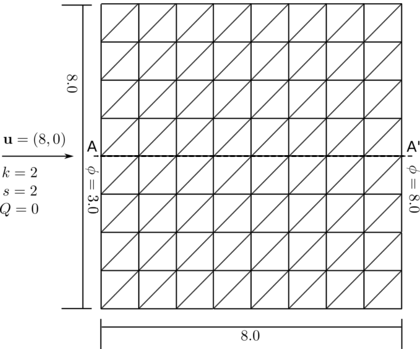Figure 1: Transient advection-absorption problem. Square domain with linear velocity and zero source.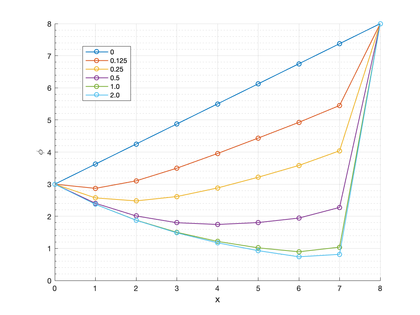Figure 2: Transient advection-absorption problem. Transient FIC-FEM solution obtained with a structured mesh of $2\times 8\times 8$ three-noded triangles. The transient solutions are plotted along line A-A' at times 0.0 s, 0.125 s, 0.25 s, 0.5 s, 1 s and 2 s.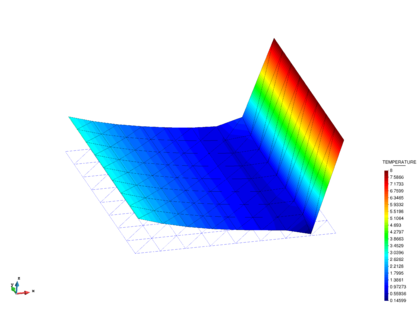Figure 3: Transient advection-diffusion-absorption problem. Solution obtained at t = 2 s.

### 7.2 Skewed flow is a square domain with non-uniform boundary conditions

The domain is ${\textstyle (x,y)=[0,1]\times [0,1]}$. The problem data is: ${\textstyle {\boldsymbol {v}}=[-5\cdot {10}^{6},-9\cdot {10}^{6}]^{T}}$, ${\textstyle k=1}$, ${\textstyle \rho c=1}$, ${\textstyle s=0}$ and ${\textstyle Q=0}$. The transported variable is again the temperature. The boundary conditions are: ${\textstyle \phi =1}$ on (${\textstyle x=1,y>0.7)\cup (x<1,y=1)}$, ${\textstyle \phi =0.5}$ at (${\textstyle x=1,y=0.7}$) and ${\textstyle \phi =0}$ on the rest of the boundary (fig:SC_scheme). The transient solution was obtained using the implicit scheme here presented with ${\textstyle \theta =0.8}$ and a time step of ${\textstyle \Delta t=1}$e-09 s. Convergence within each time step was found in 2-3 iterations. The solution develops an exponential boundary layer at the outflow boundary and an internal characteristic layer (parabolic) which is skewed to the mesh and the boundary. Both layers are subgrid phenomena for the considered mesh resolution. Both the exponential and characteristic layers are reproduced in the FIC-FEM solution without spurious oscillations near the layers. The FIC-FEM result of the evolution of the solution in time towards steady state are shown in fig:SC_results. Figure 6 shows a perspective view of the quasi steady-state solution for ${\textstyle t=2}$e-07s. The steady-state solution agrees with that reported in [51,55,62].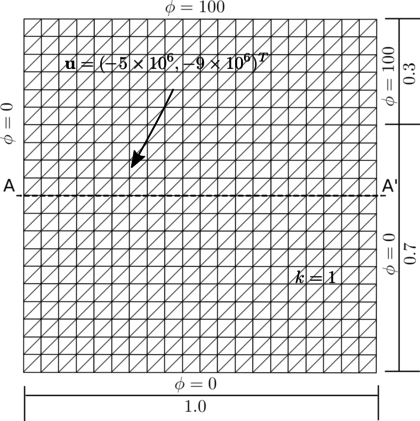Figure 4: Square domain with non-uniform Dirichlet conditions, downwards diagonal velocity and zero source. Mesh of $2\times 20\times 20$ three-noded triangles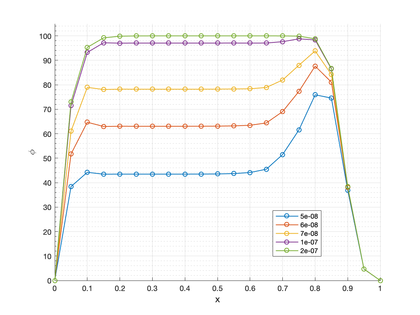Figure 5: Square domain with non-uniform Dirichlet conditions. FIC-FEM solution along line A-A'. The transient solutions are plotted at times 5e-08 s, 6e-08 s, 7e-08 s, 1e-07 s and 2e-07 s.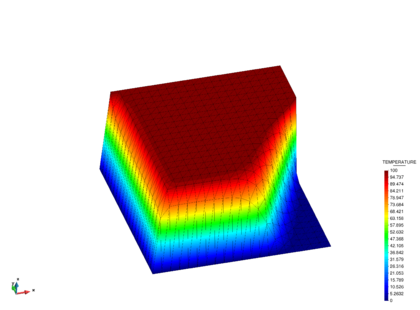Figure 6: Square domain with non-uniform Dirichlet conditions. Solution at time 2e-07 s.

### 7.3 Uniform advection problem with constant source

We study the uniform advection of a temperature field with a constant source term. The problem data is: ${\textstyle {\boldsymbol {v}}=[1,0]^{T}}$, ${\textstyle k=10^{-8}}$, ${\textstyle \rho c=1}$, ${\textstyle s=0}$ and ${\textstyle Q=1}$. The homogeneous boundary condition ${\textstyle \phi =0}$ is imposed everywhere as shown in fig:Q_source_scheme. The solution develops an exponential layer at the outflow boundary ${\textstyle x=1}$ and parabolic layers at the boundaries ${\textstyle y=0}$ and ${\textstyle y=1}$. The FIC-FEM results of the transient solution towards steady-state are shown in fig:Q_source_results. A perspective of the quasi steady-state solution at time ${\textstyle t=1.5s}$ is shown in Figure 9. Both layers are subgrid phenomena for the considered mesh resolution. The transient solution was obtained iteratively using the implicit scheme presented in the paper with ${\textstyle \theta =0.8}$ and a time step of ${\textstyle \Delta t=0.005s}$. Convergence within each time step was found in 2-3 iterations. The steady-state solution agrees with that reported in .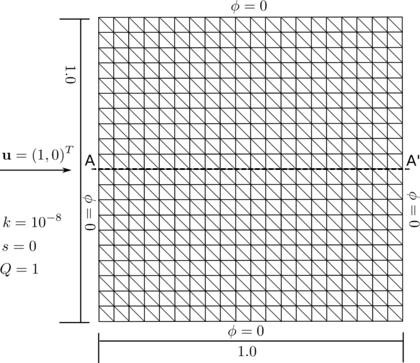Figure 7: Square domain with linear velocity and constant source. Structured mesh of $2\times 20\times 20$ three node triangles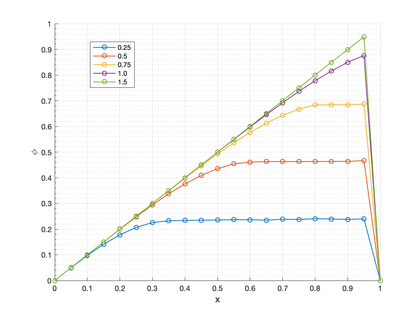Figure 8: Square domain with linear velocity and constant source. FIC solution obtained along line A-A'. The transient solutions are plotted at times 0.25 s, 0.5 s, 0.75 s, 1 s and 1.5 s.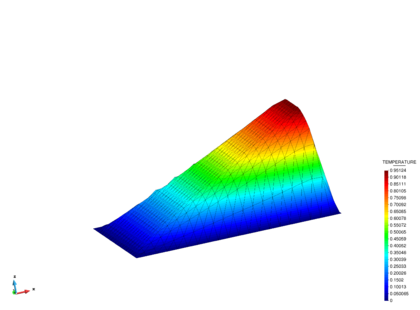Figure 9: Square domain with linear velocity and constant source. Solution at time 1.5 s.

### 7.4 Advective-diffusive transport of the temperature in a rectangular domain with Neumann and non-uniform Dirichlet conditions, rotational velocity field and zero source

The rectangular domain of sides 1 ${\textstyle \times }$ 2 units and the boundary conditions are shown in fig:rotational_scheme. The rotational velocity field is defined as ${\textstyle \mathbf {u} =10^{4}\times [y(1-x^{2}),-x(1-y^{2})]^{T}}$. A unit isotropic diffusion is assumed. The value of the temperature (${\textstyle \phi }$) is prescribed at part of the domain sides as shown in fig:rotational_scheme. In the rest of the sides, the Neumann condition (${\textstyle q_{n}^{p}=0}$) is imposed. The problem was solved with the uniform mesh of 3-noded triangles shown in fig:rotational_scheme. The correct solution is a uniform plateau of ${\textstyle \phi =100}$ with a boundary layer at the right hand side where the solution drops towards the prescribed value of ${\textstyle \phi =0}$ and a circular sharp gradient region around the lower circular zone where the solution takes a zero value. The FIC-FEM results of the transient solution towards steady-state are shown in fig:rotational_results. A perspective of the quasi steady-state solution at time ${\textstyle t=1}$e-03s is shown in Figure 12. The transient solution was obtained using the implicit scheme presented in this work with ${\textstyle \theta =0.8}$ and a time step of ${\textstyle \Delta t=1}$e-05s. Convergence within each time step was found in 2-3 iterations. The steady-state solution agrees with that reported in .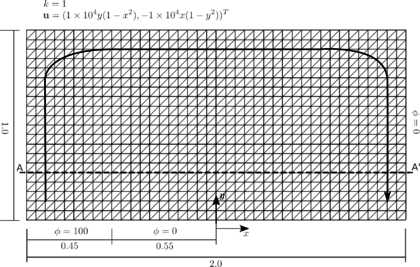Figure 10: Advective-diffusive transport of the temperature in a rectangular domain with Neumann and non-uniform Dirichlet conditions, rotational velocity and zero source. Structured mesh of 2 x 40 x 20 three-noded triangles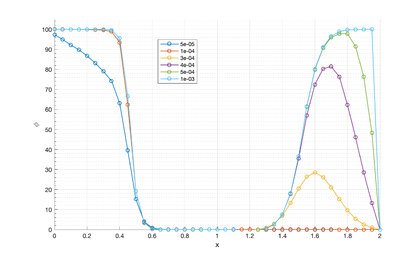Figure 11: FIC solution of the problem in Figure 10. The transient solutions are plotted along line A-A' at times 5e-05 s, 1e-04 s, 3e-04 s, 4e-04, 5e-04 and 1e-03 s.

### 7.5 Pure convection of a temperature plateau

We study the pure convection of an initial temperature plateau. The domain dimensions are ${\textstyle (x,y)=[0,1]\times [0,1]}$. The problem data is: ${\textstyle {\boldsymbol {v}}=[1,0]^{T}}$, ${\textstyle k=0}$, ${\textstyle s=0}$, ${\textstyle \rho c=1}$ and ${\textstyle Q=0}$. The homogeneous boundary condition ${\textstyle \phi =0}$ is imposed at the left wall as shown in fig:explicit_wave_scheme. The temperature plateau is generated by imposing a value of the temperature ${\textstyle \phi =1.0}$ from ${\textstyle x=0.1}$ to ${\textstyle x=0.2}$. The problem has been solved using a regular mesh of ${\textstyle 50\times 50}$ four-noded quadrilateral elements. Figures 14 and 15 show that the initial solution maintains its shape through the domain. The transient solution was obtained using an explicit scheme and a time step of ${\textstyle \Delta t=0.2s}$, giving an element Courant number of ${\textstyle C=1}$.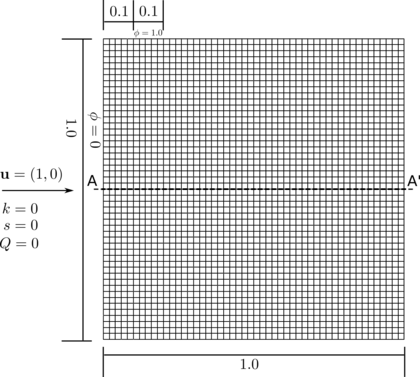Figure 13: Pure convection of a temperature plateau in a square domain with horizontal velocity. Structured mesh of 50 x 50 4-noded quadrilaterals.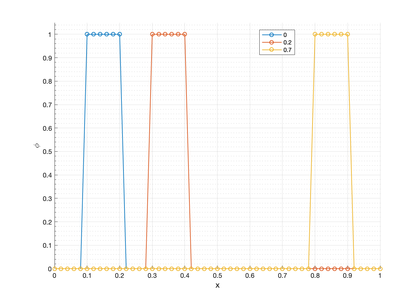Figure 14: Pure convection of a temperature plateau. FIC-FEM solution plotted along line A-A' at times 0 s, 0.2 s and 0.7 s.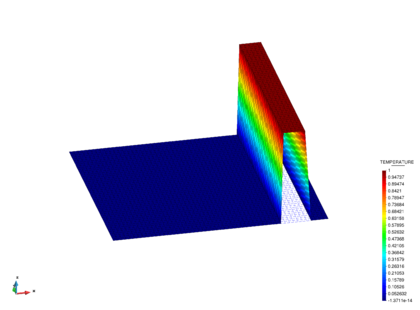Figure 15: Pure convective case. FIC-FEM solution at time 0.7 s.

### 7.6 Advection-diffusion of a concentration field

In this problem we study the advection and diffusion of a concentration that is instantaneously released as a Dirac source in a point of a stream. This problem is generally known as the plume transport problem .

The domain dimensions are ${\textstyle (x,y)=[0,35]\times [0,10]}$. The problem data is: ${\textstyle {\boldsymbol {v}}=[1,0]^{T}}$, ${\textstyle \rho c=1}$, ${\textstyle k=0.1}$, ${\textstyle s=0}$ and ${\textstyle Q=0}$. The homogeneous boundary condition ${\textstyle \phi =0}$ is imposed on the left wall as shown in fig:plume_scheme. The value ${\textstyle \phi _{0}=1000}$ is imposed as a initial value of the concentration at the node ${\textstyle (x,y)=(2,5)}$.

The analytical solution for this 2D problem is 

 $\phi (x,y,t)={\frac {\phi _{0}}{L_{3}4\pi Dt}}e^{-A}$
(48.a)

with

 $A=-{\frac {1}{4Dt}}\left\{[x_{1}-(x_{1}^{0}+v_{1}t)]^{2}+[x_{2}-(x_{2}^{0}+v_{2}t)]^{2}\right\}$
(48.b)

In Eqs.7.6 ${\textstyle \phi }$ is the initial value of the concentration that is dropped at the point with coordinates ${\textstyle {\boldsymbol {x}}^{0}=[x_{1}^{0},x_{2}^{0}]^{T}}$, ${\textstyle L_{3}}$ is the vertical dimension of the analysis domain (in this problem we have taken ${\textstyle L_{3}=1}$), ${\textstyle v_{1}}$ and ${\textstyle v_{2}}$ are the horizontal and vertical components of the velocity vector, respectively and ${\textstyle D={\frac {K}{\rho c}}}$ where ${\textstyle K}$ is the average diffusion defined in Section 2.1.

The transient solution was obtained using an implicit scheme with ${\textstyle \theta =0.5}$ and a time step of ${\textstyle \Delta t=0.25s}$, giving a Courant number of ${\textstyle C=0.5}$. We highlight that the shock-capturing terms were not taken into account for solving this problem. The use of the consistent form of the mass matrix was essential for obtaining good results for this problem. fig:plume_results_1 shows how the solution is advected and diffuses as time increases. The numerical results for the distribution of the contraction along line A-A' are in agreement with the analytical solution shown in fig:plume_results_2. The graphs are taken along the A-A' line, which goes from (0,5) to (10,5). A comparison of the contours of the numerical and analytical distributions of the concentration at ${\textstyle t=15s}$ can be seen in Figures 19 and 20, respectively.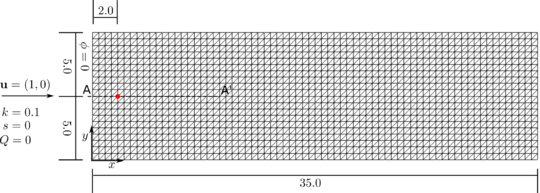Figure 16: Advection-diffusion of a concentration field. Rectangular domain with horizontal velocity. Structured mesh of 2 x 70 x 20 three-noded triangles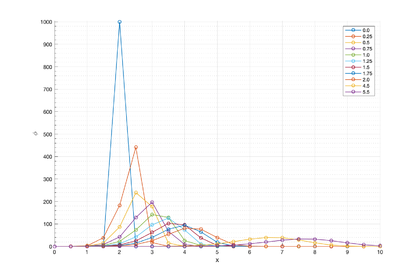Figure 17: Advection-diffusion of a concentration field. FIC-FEM solution along line A-A' obtained with the mesh of Figure 16. Structured mesh of 2 x 70 x 20 three-noded triangles. Solution at times 0 s, 0.25 s, 0.5 s, 0.75 s, 1.0 s, 1.25 s, 1.5 s, 1.75 s, 2.0 s, 4.5 s and 5.5 s.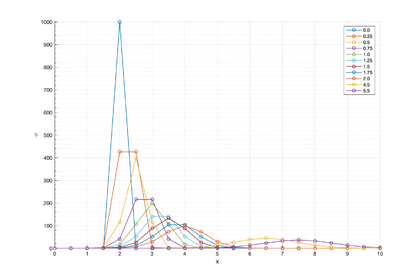Figure 18: Advection-diffusion of a concentration field. Analytical solution along line A-A' plotted on the structured mesh of Figure 16. Solution at times 0 s, 0.25 s, 0.5 s, 0.75 s, 1.0 s, 1.25 s, 1.5 s, 1.75 s, 2.0 s, 4.5 s and 5.5 s.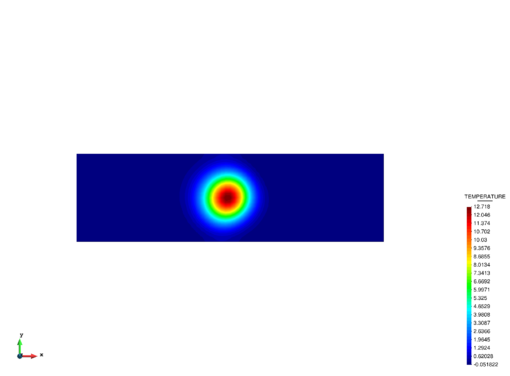Figure 19: Advection-diffusion of a concentration field. Contours of the FIC-FEM results at t = 15s.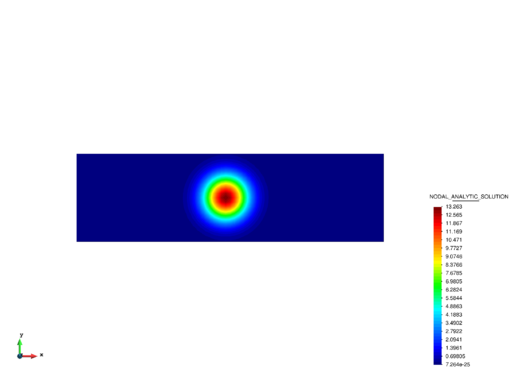Figure 20: Advection-diffusion of a concentration field. Contours of the analytical results at t = 15s.

## 8. Concluding remarks

In this work we have extended the steady-state multidimensional FIC-FEM formulation reported in  to the transient case. The stabilized variational expression has the standard residual form typical of the FIC-FEM procedure. The stabilized terms introduced by the FIC approach depend on a characteristic element length and two stabilization parameters which expression is obtained as an extension of the steady-state forms presented in . A shock capturing term is introduced to account for the Gibbs oscillations across internal/boundary layers.

The good behavior of the FIC-FEM formulation has been verified for transient advection-diffusion-absorption problems with numerical solutions evolving to a steady-state, as well as to two fully transient problems.

The numerical results were obtained in most cases using an implicit scheme. An exception is the pure convection problem (Section 7.5) that was solved with an explicit scheme and a Courant number of ${\textstyle C=1}$. The shock capturing terms were not taken into account in the solution of the fully transient problems (Sections 7.5 and 7.6). We also remark the importance of using a consistent stabilized mass matrix in the concentration field example.

## Acknowledgements

This research was partially funded by the PRECISE project (BIA2017-83805-R) of the Natural Research Plan of the Spanish Government. Support for this work was also provided from the Office for Naval Research Global (ONRG) of the US Navy through the NICE-SHIP project. We also acknowledge the financial support of the CERCA programme of the Generalitat de Catalunya.

### Document informationPublished on 01/01/2020

DOI: 10.1016/j.cma.2020.112984

### Document Score0

Views 17
Recommendations 0